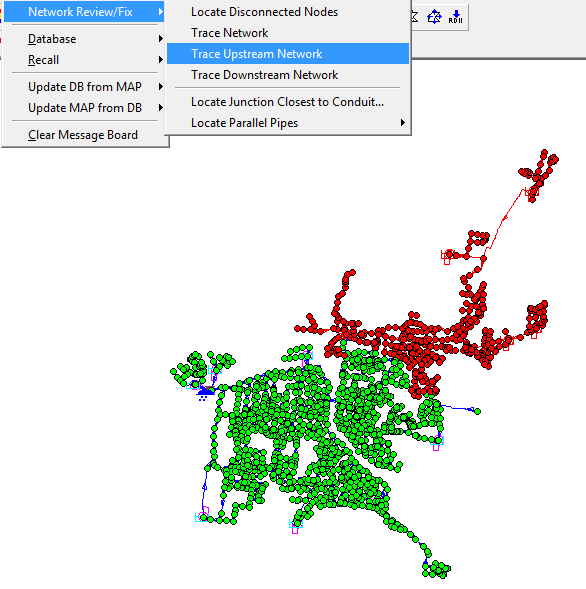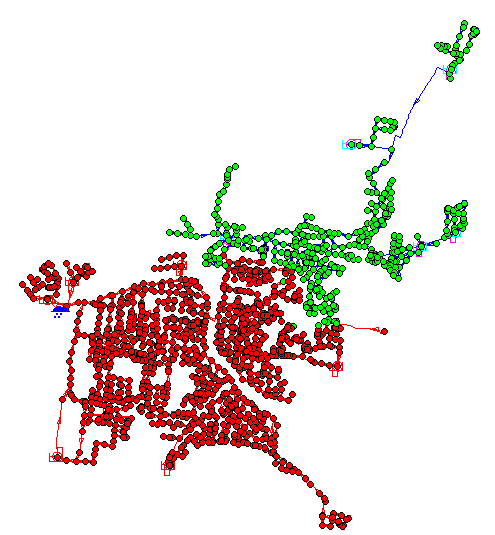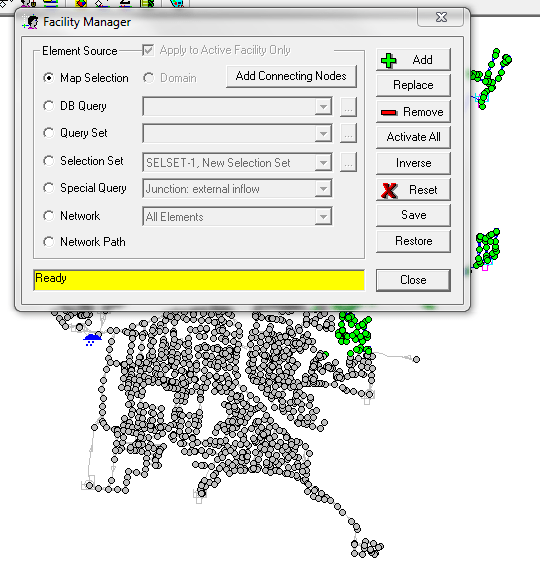# How to Easily Make a Smaller Model in InfoSWMM Using Trace Upstream Network

Subject:   How to Easily Make a Smaller Model in InfoSWMM Using Trace Upstream Network

Step 1.  Use the Trace Upstream Network command to find all of the network above the node of interest (Figure 1).

Step 2.  Use the Trace Downstream Network Command to find all of the network below the node of interest and place it in the Doman (Figure 2).

Step 3.  Use Facility Manager to make the Lower Network in the Domain Inactive (Figure 3).

Step 4.  Change the Node of Interest from a Manhole to an OutfallFigure 1.  Trace Upstream NetworkFigure 2.  Put the lower Section of the Network in a DomainFigure 3.  Use Facility Manager to make the Lower Network in the Domain Inactive

Translate »